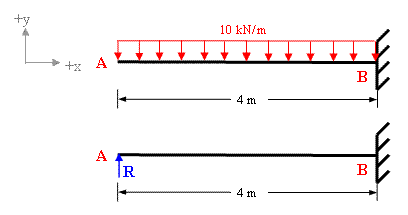Solving indeterminate beam by method of consistent deformation

Problem 7-1

The propped cantilever with applied loading is shown in figure 7-1(a). Find the support reactions and draw  Bending moment diagram.Figure 7-1(a)

Solution:

The free body diagram of this structure in fig 7-1(b) shows that  the given propped cantilever has 3 support reactions (Ay, By, MB)  whereas there are only 2 equations of equilibrium (ΣFy = 0 and ΣMz = 0) available for this structure. Therefore it is statically indeterminate of degree one.Figure 7-1(b)

To solve for the unknown reaction of this structure we need one more equation which can be obtained by compatibility. It is evident that deflection at A is zero. Considering Ay as redundant R and applying principle of superposition we get;

Total deflection at A is equal to the sum of deflection due to applied loading and deflection due to redundant R (refer to figure 7-1(c).Figure 7-1(c)

Deflection at A due to applied loading = wL4 /8EI = (10 x 44)/8EI

Deflection at A due to redundant R = (R x 43)/3EI

Taking downward deflection as negative and upward as positive and applying principle of superposition.

ΣδA = (R x 43)/3EI  - (10 x 44)/8EI

But ΣδA = 0 as the support A is a rigid support.

Therefore (R x 43)/3EI  - (10 x 44)/8EI  = 0

R = {(10 x 44)/8EI} / { ( 43)/3EI}

Hence R = 15 kN

Now we can easily determine the other reactions

By  = 10 x 4 - 15 = 25 kN

MB= 15x4 - 10x4x2 = - 20 kNm               (clockwise)

The resultant bending moment diagram shown in figure 7-1(d) is drawn by superimposing the negative moment diagram over the positive moment diagram. The positive moment diagram will be parabolic (due to udl) whereas negative moment diagram will be a straight line (due to point load)Fig 7-1(d|)

The resultant BMD shows that this beam will have a point of contra-flexure (also known as point of inflection) which can be determined in a simple way by writing the equation of bending moment at a distance 'x' and equating it to zero because the bending moment is equal to zero at the point of contra-flexure.

Mx = 15x -10x2 /2 = 0

Therefore x = 3m from the prop A,

It is clear from the resultant BMD that the maximum bending moment value is greatly reduced.

You can also use our deflection calculator for calculating deflection to solve this problem

##### Please visit the following links of solved examples for Indeterminate Structures

You can also visit other solved examples

#### Excellent Calculators

Stress Transformation Calculator
Calculate Principal Stress, Maximum shear stress and the their planes

To determine Absolute Max. B.M. due to moving loads.

Bending Moment Calculator
Calculate bending moment & shear force for simply supported beam

Moment of Inertia Calculator
Calculate moment of inertia of plane sections e.g. channel, angle, tee etc.

Reinforced Concrete Calculator
Calculate the strength of Reinforced concrete beam

Moment Distribution Calculator
Solving indeterminate beams

Deflection & Slope Calculator
Calculate deflection and slope of simply supported beam for many load cases

Fixed Beam Calculator
Calculation tool for beanding moment and shear force for Fixed Beam for many load cases

BM & SF Calculator for Cantilever
Calculate SF & BM for Cantilever

Deflection & Slope Calculator for Cantilever
For many load cases of Cantilever

Overhanging beam calculator
For SF & BM of many load cases of overhanging beam

Civil Engineering Quiz
Test your knowledge on different topics of Civil Engineering

Research Papers
Research Papers, Thesis and Dissertation

List of skyscrapers of the world
Containing Tall buildings worldwide

Forthcoming conferences
Containing List of civil engineering conferences, seminar and workshops

Profile of Civil Engineers
Get to know about distinguished Civil Engineers

Professional Societies
Worldwide Civil Engineers Professional Societies